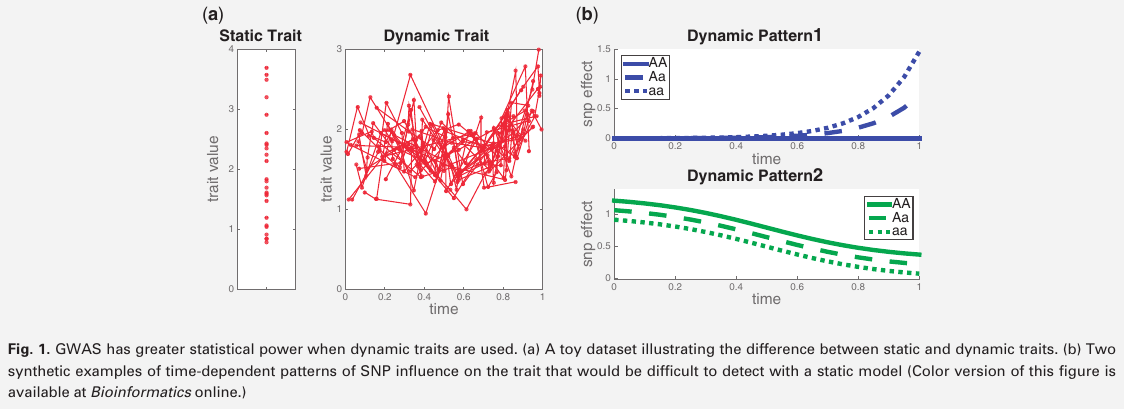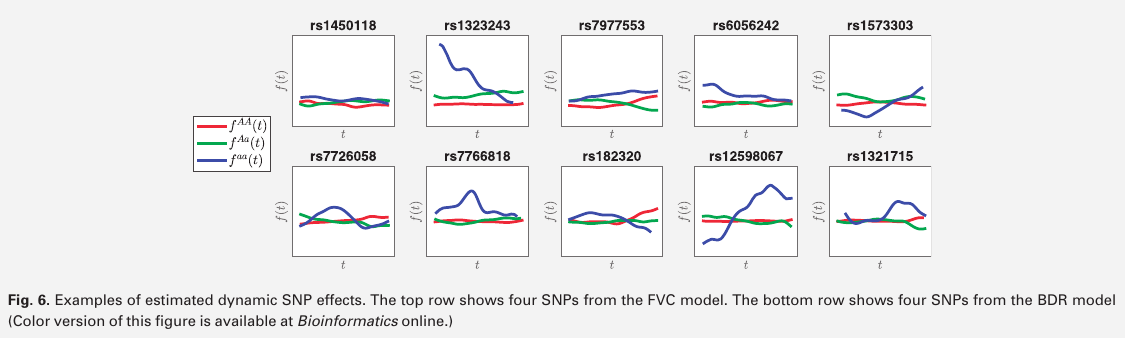# Time-varying Group Sparse Additive Model for GWAS

##### Posted on Jun 11, 2023 (Update: Jul 27, 2023)
Tags: GWAS, Time-varying
• most existing GWAS methodologies are still limited to the use of static phenotypes measured at a single point.
• the paper proposes a new method for association mapping that considers dynamic phenotypes measured at a sequence of time points.
• Time-Varying Group Sparse Additive Models (TV-GroupSpAM) for high-dimensional, functional regression

• fGWAS: performing GWAS of dynamic traits, construct a separate model to estimate the smooth, time-varying influence of each SNP on the phenotype.
• once the mean effects have been estimated for each genotype at each time point, a hypothesis test is performed to determine whether the SNP has any additive or dominant effect on the trait.
• the principal drawback is that it is inappropriate for modeling complex traits that arise from interactions between genetic effect at different loci.
• the paper introduce a new penalized multivariate regression approach for GWAS of dynamic quantitative traits, in which the phenotype is modeled as a sum of nonparametric, time-varying SNP effects
$Y(T) = f_0(T) + \sum_{j=1}^pf_j(T, X_j) + w(T) = f_0(T) + \sum_{j=1}^p\sum_{g=0}^2 f_j^g(T)X_j^g + w(T)$Published in categories Note